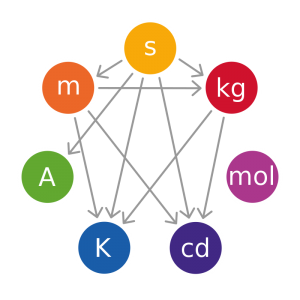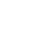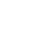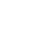# Mass and Weight

Physics is the science that studies motion and matter and how both work with forces and energy. It is a really big subject that has a lot of different “branches” of scientific study that include motion, sound, waves, electricity, light, sound, and even astronomy.

This science covers the tiniest particles and atoms all the way to the largest objects in the universe.When scientists talk about the “mass” of an object they are really referring to how much matter is in the object. It’s a total of all of the atoms, the density or how compact the atoms are, and the type of atoms in the object.### Figuring out the Measurement of Mass

Scientists have decided that mass is usually measured in “kg” or kilograms, but figuring out the mass of an object is kind of complex. They use two common ways to get an objects mass:

• Inertial mass is figuring out how much that object resists acceleration. An example of this might be to put two objects in the same conditions and push them with the same amount of force. The object that has the lowest mass will accelerate faster than the other one.
• Gravitation mass is measuring how much gravity one object exerts on other objects or how much gravity an object experiences from another object.### The difference between weight and mass?

The weight of an object is different from its mass. Weight is the measurement of the gravitation force on an object and it can change based on where it’s located. An object’s mass will never change.

An example of the weight change can be someone on Earth that weighs 100 lbs but would be weightless in outer space or weigh 38 lbs on Mars. The mass of that person would always be the same.

### Size and mass are different

We sometimes calls “size” volume. This is because the type of molecules or atoms that make up an object also determine their “density” which helps to figure out its mass.

An example of this is a helium-filled balloon. It has a lot less mass than the same sized item that is made of solid steel. It shows that the size of an object doesn’t determine its weight.

### The weird law of conservation of mass

One of the laws that seems odd but makes a lot of sense is the “law of conservation of mass.” This says that the mass of a closed system must remain constant over time, even when changes are made to the objects within the system.

An example of this would be to add sugar to water and then mix the contents. When you weigh the mixture the weight shows the weight of both the sugar and the water, even though it looks like the sugar dissolved in the water.

## Interesting Facts about weight and mass:

• The word “mass” originates from “maza,” which is a Greek word that means “lump of dough.”
• The gravity on Earth changes, depending upon where you are. This means that you could weigh more or less by as much as 0.5% in some places.
• Plato was a Greek philosopher who said that weight was the “natural tendency of objects to seek their kin.”
• Scientists have estimated that total mass of the universe at between 1052kg and 1053 kg
Some meteorites that have been found are very small, but because they are made up of iron that is very dense, they can weigh a lot.
• The Earth has six times more gravity than the moon and that means an object weights 1/6thof that on Earth.
• The mass of our Earth is six trillion trillion kilograms!
• Outer space has no gravity and that’s why they call objects in outer space “weightless.”

### Q&A

What is the measurement that scientists use for mass?
kg or kilograms

Are mass and weight the same?
no

What are the two methods used by scientists to figure out an objects mass?
inertial mass and gravitational mass

Which always remains the same: mass or weight?
mass

What is the definition of weight?
the measurement of the gravitation force on an object and it can change based on where it’s located

What is another word sometimes used for size?
volume

•History
•Science
•Geography
•Biography
•US History